##### Partitions, Compositions, and Young Tableaux

A partition of a positive integer $n$ is a set of $k$ strictly positive integers that sum up to $n$. A composition of $n$ is a particular arrangement of nonŁnegative integers that sum up to $n$. A Young tableaux is a structure of integers $1, ..., n$ where the number of elements in each row is defined by an integer partition of $n$. Further, the elements of each row and column are in increasing order, and the rows are left justified. These three related combinatorial objects have a host of interesting applications and properties.

Here are the eleven partitions of 6. Observe that they are given in reverse lexicographic order.

`Partitions`
`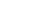`

Although the number of partitions grows exponentially, it does so more slowly than permutations or subsets, so complete tables can be generated for larger values of $n$.

`Length[Partitions]`
``

Ferrers diagrams represent partitions as patterns of dots. They provide a useful tool for visualizing partitions, because moving the dots around provides a mechanism for proving bijections between classes of partitions. Here we construct a random partition of 100.

`FerrersDiagram[RandomPartition]`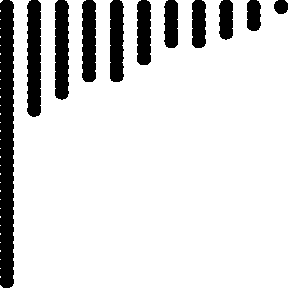`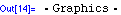`

Here every composition of 6 into 3 parts is generated exactly once.

`Compositions[6,3]`
`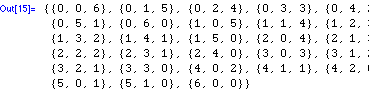`

The list of tableaux of shape $\left\{ 3, 2, 1 \right\}$ illustrates the amount of freedom available to tableaux structures. The smallest element is always in the upper leftŁhand corner, but the largest element is free to be the rightmost position of the last row defined by the distinct parts of the partition.

`Tableaux[{3,2,1}]`
`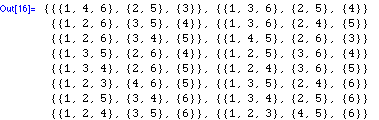`

By iterating through the different integer partitions as shapes, all tableaux of a particular size can be constructed.

`Tableaux`
`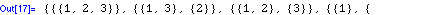`

The hook length formula can be used to count the number of tableaux for any shape. Using the hook length formula over all partitions of $n$ computes the number of tableaux on $n$ elements.

`NumberOfTableaux`
`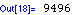`

Each of the 117,123,756,750 tableaux of this shape will be selected with equal likelihood.

`TableForm[ RandomTableau[{6,5,5,4,3,2}] ]`
`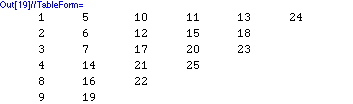`

A pigeonhole result states that any sequence of $n^2 + 1$ distinct integers must contain either an increasing or a decreasing scattered subsequence of length $n + 1$.

`LongestIncreasingSubsequence[ RandomPermutation ]`
`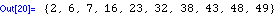`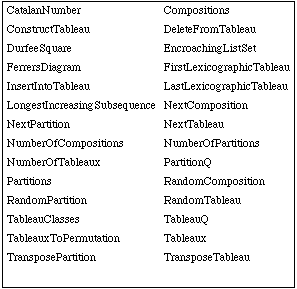Combinatorica functions for partitions, compositions and Young tableaux.